# PROBLEM #6 The equilibrium price of cars in Boston in an unregulated automobile market is \$25,000...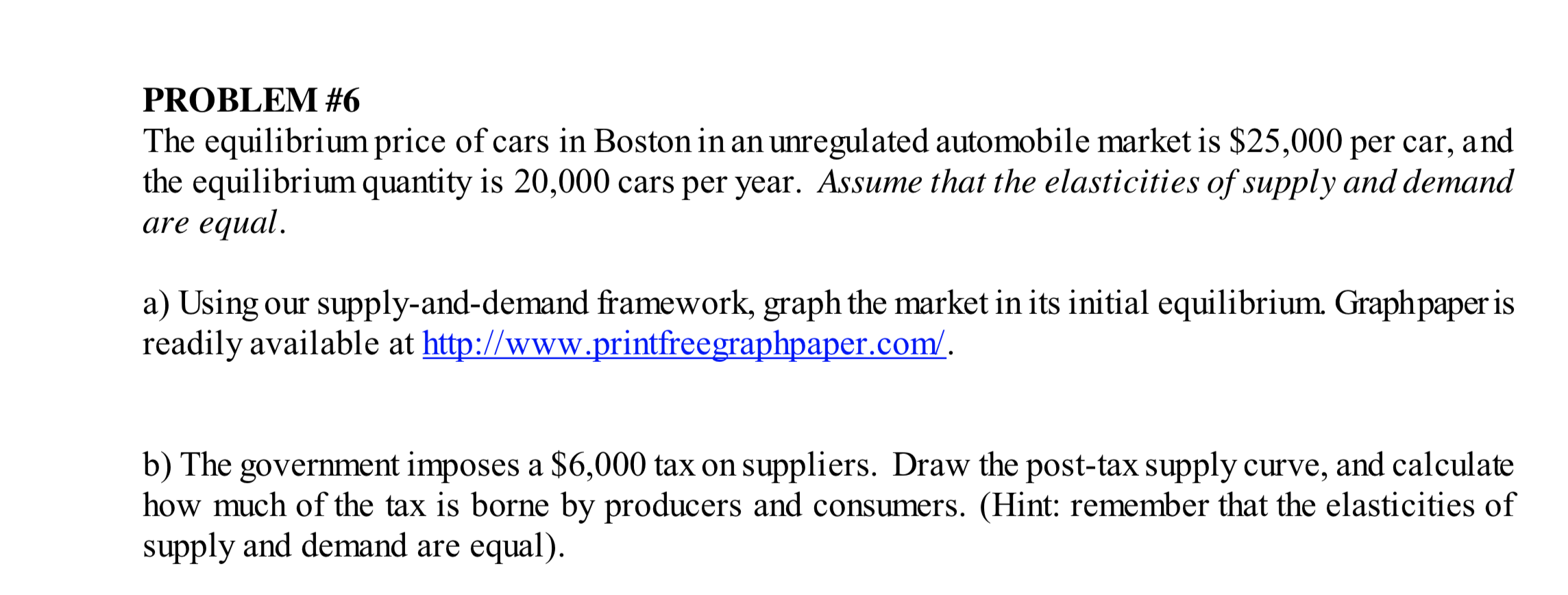PROBLEM #6 The equilibrium price of cars in Boston in an unregulated automobile market is \$25,000 per car, and the equilibrium quantity is 20,000 cars per year. Assume that the elasticities of supply and demand are equal. a) Using our supply-and-demand framework, graph the market in its initial equilibrium. Graphpaper is readily available at http://www.printfreegraphpaper.com/. b) The government imposes a \$6,000 tax on suppliers. Draw the post-tax supply curve, and calculate how much of the tax is borne by producers and consumers. (Hint: remember that the elasticities of supply and demand are equal).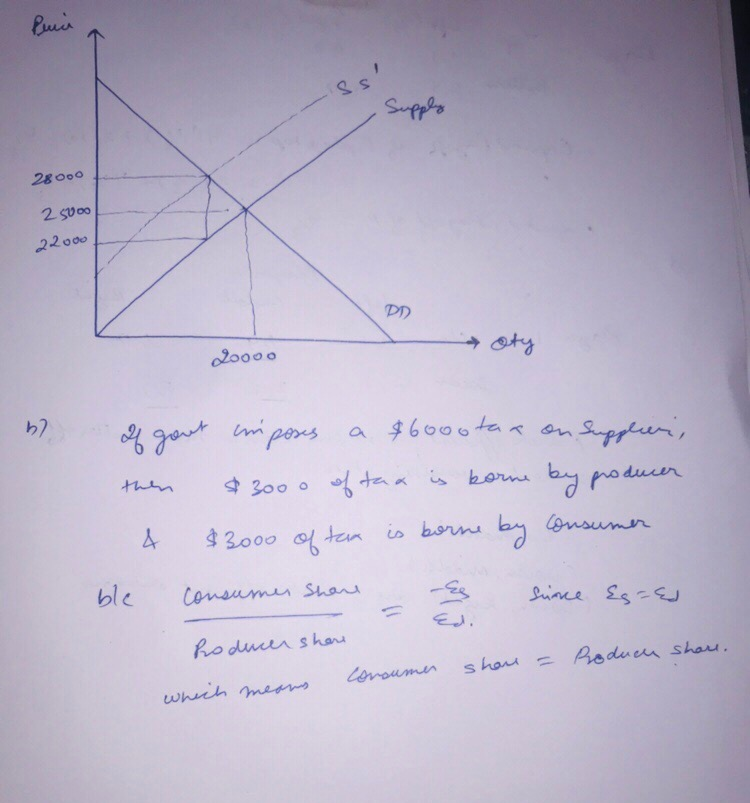##### Add Answer of: PROBLEM #6 The equilibrium price of cars in Boston in an unregulated automobile market is \$25,000...
Similar Homework Help Questions
• ### 3. (20%) Consider the market was unregulated, with an equilibrium price of \$200, and a quantity of 20,000 The suppl...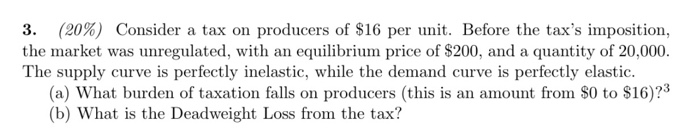3. (20%) Consider the market was unregulated, with an equilibrium price of \$200, and a quantity of 20,000 The supply curve is perfectly inelastic, while the demand curve is perfectly elastic. (a) What burden of taxation falls on producers (this is an amount from \$0 to \$16)?3 (b) What is the Deadweight Loss from the tax? a tax on producers of \$16 per unit. Before the tax's imposition, 3. (20%) Consider the market was unregulated, with an equilibrium price of...

• ### 5. TAXES/SUBSIDIES, AND OTHER GOVERNMENT REGULATIONS 1. Consider the demand and supply for bubbly water in...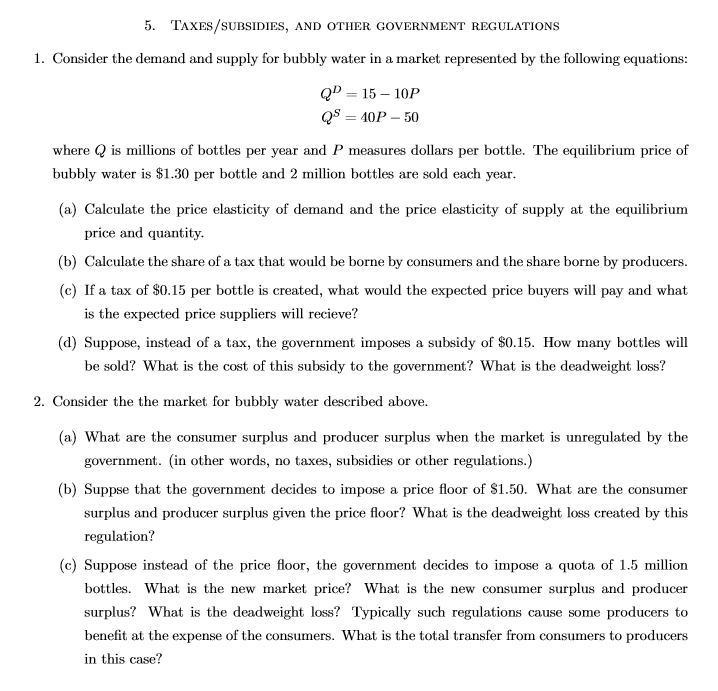5. TAXES/SUBSIDIES, AND OTHER GOVERNMENT REGULATIONS 1. Consider the demand and supply for bubbly water in a market represented by the following equations: QD = 15 - 10P QS = 40P - 50 where Q is millions of bottles per year and P measures dollars per bottle. The equilibrium price of bubbly water is \$1.30 per bottle and 2 million bottles are sold each year. (a) Calculate the price elasticity of demand and the price elasticity of supply at the...

• ### Suppose that demand and supply functions for good X are: QD=90-10P (P=9-0.1QD) QS=20P-6 (P=0.3+0.05QS) a. Graph...

Suppose that demand and supply functions for good X are: QD=90-10P (P=9-0.1QD) QS=20P-6 (P=0.3+0.05QS) a. Graph this situation. b. What is the equilibrium price and quantity in the market for good X? c. What is consumers surplus? Producers surplus? d. Suppose the government imposes a per unit tax on good X equal to 1 dollar (per unit). What is the new equilibrium price and quantity? How much revenue would this tax raise for the government? What is consumers surplus? Producers...

• ### When the government imposes a price ceiling set below the market equilibrium price, which of the...

When the government imposes a price ceiling set below the market equilibrium price, which of the following will occur?A. Producers will increase the quantity in supplyB. A decrease in demand and a increase in supplyC. An increase in both demand and supplyD. A decrease in both demand and supply

• ### 1. Suppose market demand for oranges is given by QD = 500 - 10P where Qp...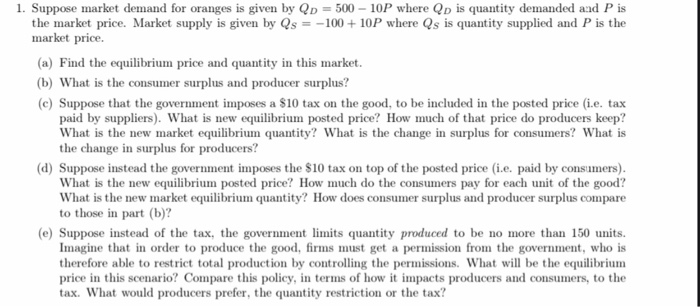1. Suppose market demand for oranges is given by QD = 500 - 10P where Qp is quantity demanded and P is the market price. Market supply is given by Qs = -100 + 10P where Qs is quantity supplied and P is the market price. (a) Find the equilibrium price and quantity in this market. (b) What is the consumer surplus and producer surplus? (C) Suppose that the government imposes a \$10 tax on the good, to be included...

• ### The graphs show the market for bags of potato chips, which is currently at an equilibrium...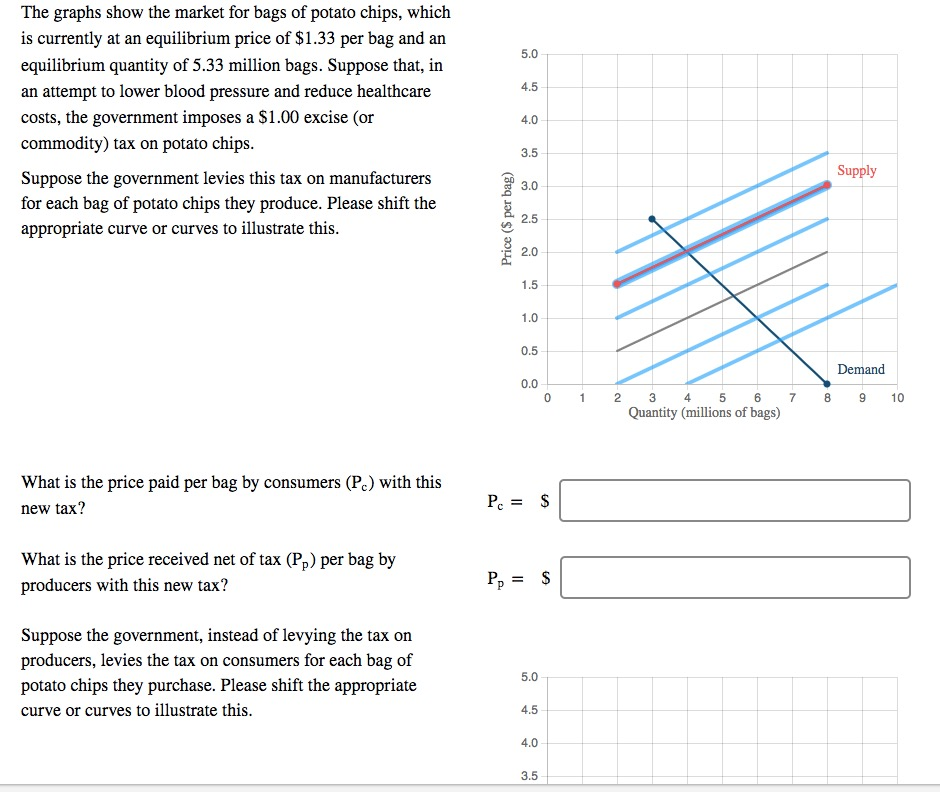The graphs show the market for bags of potato chips, which is currently at an equilibrium price of \$1.33 per bag and an equilibrium quantity of 5.33 million bags. Suppose that, in an attempt to lower blood pressure and reduce healthcare costs, the government imposes a \$1.00 excise (or commodity) tax on potato chips Suppose the government levies this tax on manufacturers for each bag of potato chips they produce. Please shift the appropriate curve or curves to illustrate this...

• ### The demand and supply for automoblles In a certain country is given In the graph below....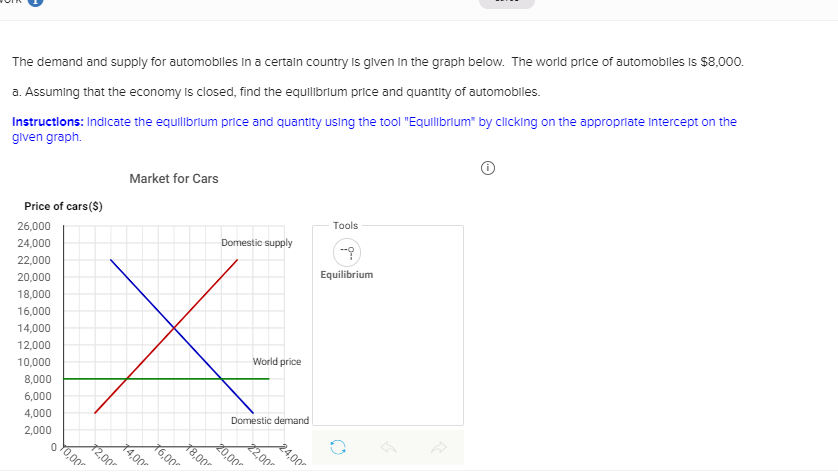The demand and supply for automoblles In a certain country is given In the graph below. The world price of automobles is \$8,000. a. Assuming that the economy Is closed, find the equilibrium price and quantity of automobles. Instructions: Indicate the equilibrium price and quantity using the tool "Equilibrium* by clicking on the appropriate Intercept on the given graph. Market for Cars Price of cars (S) 26,000 24,000 22,000 20,000 18,000 16,000 14,000 12,000 10,000 8,000 6,000 4,000 2,000 Tools...

• ### In this question, we study the consequences of trade policies on the automobile market. We assume...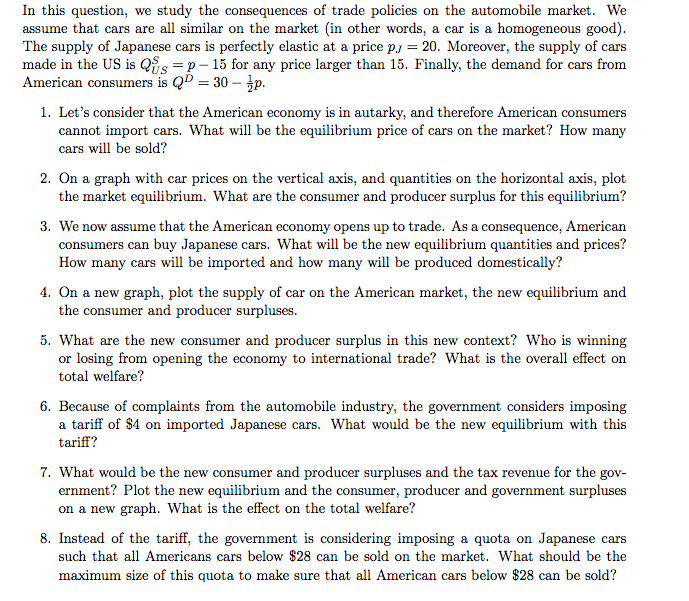In this question, we study the consequences of trade policies on the automobile market. We assume that cars are all similar on the market (in other words, a car is a homogeneous good). The supply of Japanese cars is perfectly elastic at a price pJ = 20. Moreover, the supply of cars made in the US is QSus = p − 15 for any price larger than 15. Finally, the demand for cars from American consumers is QD = 30...

• ### Problem #1

PROBLEM #1An economist, working for a public health agency, has estimated the supply and demand equations for fast-food cheeseburgers in an urban area as follows:Demand: Qd=2,450- 500PSupply: Qs=200Pa. Determine the market equilibrium price and quantity for this market.b. Calculate the price elasticities of demand and supply and, in each case,determine whether it is elastic, inelastic, or unit elastic.c. The public health agency is concerned with the rise in obesity, high bloodpressure, and diabetes, and persuades legislators to enact a \$.75...

• ### Question 1 1 pts The demand curve in a competitive market is given by P =...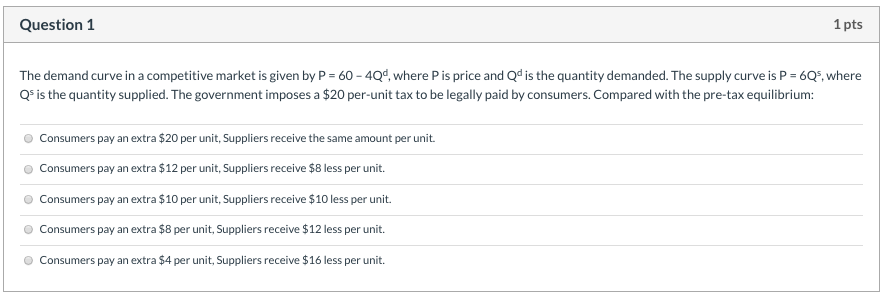Question 1 1 pts The demand curve in a competitive market is given by P = 60 - 404, where Pis price and Qd is the quantity demanded. The supply curveis P = 6Q5, where Qs is the quantity supplied. The government imposes a \$20 per-unit tax to be legally paid by consumers. Compared with the pre-tax equilibrium: Consumers pay an extra \$20 per unit, Suppliers receive the same amount per unit. Consumers pay an extra \$12 per unit, Suppliers...

Need Online Homework Help?